﻿ Design and Implementation of Controlled Zeta Converter Power Supply

### Design and Implementation of Controlled Zeta Converter Power Supply

Ali H. Ahmad, Nashwan Saleh SultanOPEN ACCESSPEER-REVIEWED

## Design and Implementation of Controlled Zeta Converter Power Supply

Ali H. Ahmad1, Nashwan Saleh Sultan2,1Department of Electrical, Eng. College, University of mosul, Mosul, Iraq

2Technical Eng.College of Mosul, Mosul, Iraq

### Abstract

This work includes Zeta voltage converter controller design and implementation. The mathematical model of the zeta converter circuit operating in the continuous conduction mode in state-space form is presented. Fuzzy Logic controller and hybrid Fuzzy Logic controller/ particle swarm optimization techniques are used to designed controller. Analysis and comparison between simulation and practical responses of open loop, close loop fuzzy logic controller and hybrid fuzzy logic/particle swarm optimization controller results are performed for different, working conditions such as sudden changes in the load resistance and reference voltages. The results show that there are significant improvement in the results for the proposed hybrid FLC/PSO control technique.

### At a glance: Figures

12345
Prev Next

• Ahmad, Ali H., and Nashwan Saleh Sultan. "Design and Implementation of Controlled Zeta Converter Power Supply." American Journal of Electrical and Electronic Engineering 2.3 (2014): 121-128.
• Ahmad, A. H. , & Sultan, N. S. (2014). Design and Implementation of Controlled Zeta Converter Power Supply. American Journal of Electrical and Electronic Engineering, 2(3), 121-128.
• Ahmad, Ali H., and Nashwan Saleh Sultan. "Design and Implementation of Controlled Zeta Converter Power Supply." American Journal of Electrical and Electronic Engineering 2, no. 3 (2014): 121-128.

 Import into BibTeX Import into EndNote Import into RefMan Import into RefWorks

### 1. Introduction

A DC-DC converter is widespread in modern portable electronic equipments and systems. The batteries are providing constant input voltage to the converter, then the converter converts it into wide range of values depending on the charge level. At low charge level, the voltage may drop below the battery voltage for continuously supplying the load with constant voltage [1, 2]. There for it needs to be regulated. There are many research works dealing with the direct voltage converters performances and their control. One of those works was that of (A. H. Ahmed ..et, al) in 2006 A.C . They designed and analyzed a regulated controller of Cuk converter using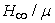technique. O. A. Taha in 2007A.C had studied CUK converter circuit performances, its designed and implement a robust controller for the cuk converter usingsynthesis technique. He studied the effects of CUK converter parameters changes on the circuit stability. S. S.Sabri in 2008 A.C, he studied the CUK converter circuit performance and designed controller for it using Fuzzy Logic Controller based genetic algorithm to improve its performance . E. Vuthchhay and C. Bunlaksanusorn in 2010A.C . They studied zeta converter circuit performance, Modeling and Control of the converter performance. R. Suresh Kumar, had studied BOOST converter circuit performance and its control using PID controller. He used a particle swarm optimization technique for the design and improvement of its performance . Because of the lack of the studies about the ZETA converter circuit, the present work is dealing with the design of the Fuzzy Logic Controller and hybrid Fuzzy Logic/ Particle Swarm Optimization Controller to control converter circuit output voltage and improve its output performance.

### 2. Zeta Converter Mathematic Model

The dc-dc voltage Zeta converter is assumed to operate in the continuous conduction mode(CCM).There exist two circuit states within one switch period T. First state is when switch is turned on (DT),and another when it is turned off [(1-D)T].The general state space mathematical model of the zeta converter is given by: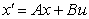(1)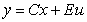(2)

Where

x: is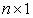state vector; A: is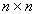system matrix; u:is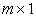input vector;

B: isinput matrix; E: is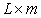matrix; y : is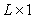output vector; C: is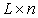output matrix;

The zeta converter circuit is shown in Figure 1. It consists of IGBT transistor as a switch, Diode, two capacitors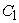and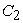, two inductors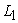and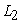with internal resistances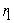and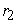respectively and load resistor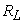. In the first mode of operation, the converter circuit(switch is ON) shown in Figure 2. During the interval (DT), the inductorsandare in charging state.

Considering :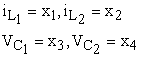Applying the Kirchhoff's voltage law to the circuit in Figure 2 and writing the voltage equations for the open loop circuit and rearranging the equations to be in the state space form. The system equations became to be: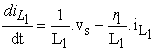(3)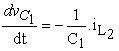(4)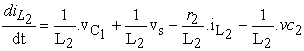(5)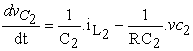(6)

In the second mode of operation (1-D)T the IGBT transistor is OFF. The converter equivalent circuit is shown as in Figure 3. In this mode the inductors (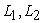) are in the discharging state.is discharging its stored energy into the capacitor, and the inductortransform energy to output section [6, 8]. Then by the same way the system equations became to be: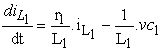(7)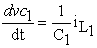(8)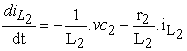(9)(10)

Using on state and off state equation, the system state space equivalent equation became to be: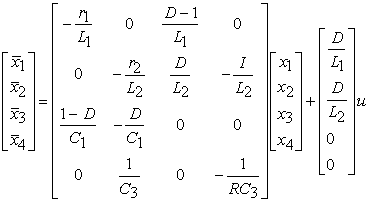(11)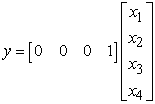(12)

The relation between input and output voltages of the zeta converter is given by: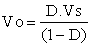(13)

### 3. Zeta Converter Circuit Design

Applying Kirchhoff's voltage law on Zeta converter circuits: first and second modes. The produced ripple inductors currents and ripple capacitors voltages are given by the equations below: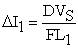(14)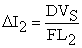(15)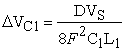(16)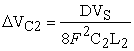(17)

Where: F is the switching frequency (F=5Khz),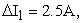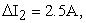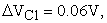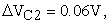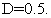From the equations above the critical values of the inductors and capacitors of the Zeta converter circuit may be introduced as the follows: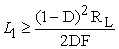(18)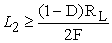(19)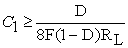(20)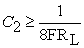(21)

Whereas the above components became to be:= 0.5mH,= 0.5mH,=900µF,= 1000µF.

### 4. Fuzzy Logic Controller Design

Fuzzy logic controller has been designed for writing its inputs as : The error e(t)and the error change ∆e(t) of the output voltage. The linguistic variables are defined as (N, NS, Z, PS, P)where N means negative, NS negative small, Z zero, PS positive small, P positive. Triangular membership functions of the fuzzy logic controller are considered. The fuzzy rules are summarized in Table 1. The surge no type of fuzzy inference engine is considered . The error range are taken between (-30 and 30) as shown in Figure 4a, and range of error change are taken between(-12 and 12) as shown in Figure 4b.

Figure 5. output voltage responses of the open loop and closed loop systems with FLC when Vref =9,12 and15 volts and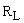(10) Ω

#### Table 1. Fuzzy Logic Controller rule base

The output voltage responces for the open loopand closed loop systems with Fuzzy Logic Controller are shown on the same graph in Figure 5 for referance voltages (9,12 and15) volts and load resistance (10) Ω.

### 5. Particle Swarm Optimization Algorithm

Particle swarm optimization technique, first developed by (J. Kennedy and R. Eberhart, 1995) as one of the modern heuristic algorithms. It was inspired by the social behavior of the bird and fish schooling and has been found to be robust in solving continuous nonlinear optimization problems [7, 9]. This algorithm is based on the following scenario: a group of birds are randomly searching for food in an area and there is only one piece of food. All birds are unaware where the food is, but they do know how far the food is at each time instant. The best and most effective strategy to find the food would be to follow the bird which is nearest to it. Based on such scenario, the PSO algorithm is used to solve the optimization problem.

In PSO, each single solution is a “bird” in the search space; this is referred to as a “particle”. The swarm ismodeled as particles inamulti-dimensional space, which have positions and velocities. These particles have two essential capabilities: their memory of their own best position and knowledge of the global best. Members of the swarm communicate good positions to each other and adjust their own position and velocity based on good positions according to following equations (22,23): [7, 10].

Let:

i to be the number of particles

j to be the number of iterations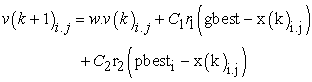(22)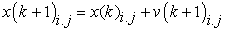(23)

Where as: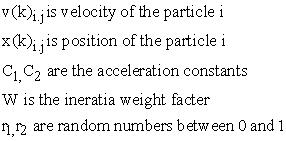Pbest is the best position of the specific particle

Gbest is the best particle of the group.

### 6. Hybrid Fuzzy Logic /Particle Swarm Optimization Controller Design

The design of an optimal fuzzy logic controller is performed using the PSO algorithms to search globally its optimal gains. The structure of the fuzzy logic controller with PSO algorithms is shown in Figure 6. The optimization algorithm is implemented by using MATLAB m-file program and linked with the system simulation program in MATLAB/SIMULINK, to check the system performance in each particle. The PSO produces the FLC controller gains which give optimal performance for the Zeta converter. The minimum absolute integral error is adopted in this work, which is given by the following equation (20).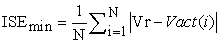(24)

When n: is number of semples considered.

The PSO technique has been programed using MATLAB M-file. PSO algorithm is used in this work to compute the fuzzy controller gains G,G1,H during the system disturbance, the error information reaches the PSO algorithm. Then, it startes to update the fuzzy gains values for mantianing the desired system performance..The hybrid FLC/ PSO flowchart is shown in Figure 7.

### 7. System Realizationz

The system is implemented practically as open loop, closed-loop with FLC and hybrid FLC/ PSO as shown in Figure 8. The open-loop voltage response when duty ratio (D=0.5555) and load resistance (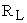= 10-40-10Ω) is shwon in Figure 9. The close loop voltage response with FLC when referance voltage (Vref=15 volt) and load resistance (= 10Ω) is shwon in Figure 10. The close loop voltage response with hybrid FLC/ PSO when referance voltage (Vref=15 volt) and load resistance (= 10Ω) is shwon in Figure 11.

Figure 9. output voltage response of the open loop when (D=0.5555) and (=10-40-10 Ω)
Figure 10. output voltage response of the closed loop system with FLC for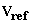15 volt and (= 10-40-10) Ω
Figure 11. output voltage response of the closed loop system withhybrid FLC/PSOfor= 15 volt and (= 10-40-10) Ω

### 8. Results and Discussion

The output voltages for the simulated openloop and closed loop systems with fuzzy logic controller are shwon in Figure 12, for referance voltages (9, 12 and 15) volt, for varying the load resistance (10-40-10)Ω at time (t=0.05-0.1-0.15 sec) respectively. The fuzzy logic controller gains are chosen by trail and error method to get the bestzeta converteroutput performance. Thisprocess needed long time. The closed loop system with the FLC/PSO controller response shown in Figure 13 has agreat improvement in it performance of the absolute itegral error was minimum. It oovercome the probem of long time mannal tunning. The output voltage of theclosed loop system with FLC had beenadaptedusing PSOtechniquewhen referance voltages (9,12 and 15) voltsand varying load resistances equal to (10-40-10Ω) as shwon in Figure 13. The closed looppractical system response with FLC/ PSO when referance voltages (9-12-15volt) and load resistance (10Ω) is shwon in Figure 14. Comparision between theoritical and practicalresults for open loop, close loop with FLC and hybrid FLC/PSO controllerfor voltage (15 volt) are shwon in the Table 2.

Figure 12. open loop system and close loop system with FLC controller responsesfor= 9,12,15 volts and= 10-40-10 Ω
Figure 13. close loop system with FLC/ PSO controller responsesfor= 9,12,15 volts and=10-40-10 Ω
Figure 14. output voltage response of the closed loop system for= 9,12,15 volts and(10) Ω.withFLC /PSO controller

#### Table 2. Comparision of the system performance for the different techniques when referance voltage (Vref=15 volts)

From Table 2 above, it is shown that the performance of the converter for open loop system has high peak overshoot, the steady state time and state steady error. The system manually optimized fuzzy controller gains response performance (P.O.S, tss and ess) have been improved. Hybrid FLC/PSO (P.O.S, tss and ess) has improvements. The realized system response performance appears verified the theoretical ones except the steady state time witch was slightly higher. Comparison of the results show that the proposed(FLC/PSO)control is the best.

### 9. Conclusion

Zeta converter circuit has been designed. Its mathematical model in state space form has been developed. The circuit output voltage response as an open-loop system has been analyzed. Two techniques has been use to regulate the output voltage of the zeta converter circuit. Fuzzy logic controller technique has been used. Hybrid fuzzy logic /particle swarm optimization technique has been proposed. The output voltage for the Zeta converter with the feedback controller and two technique has been studied. It has been seen that the response of the Zeta converter circuit with the proposed technique was better than that of FLC technique. The computation time for the FLC/PSO gains were very short. The designed circuit with the controllers was implemented. The practical results was verified the simulated results. Therefore one can conclude that the proposed method is the best one.

### References

  J. Falin, “Designing DC/DC converters based on ZETA topology”, Analog Applications Journal, Texas Instruments Incorporated, pp, 16-20, 2010.In article  D. C. Martins, “Zeta Converter with High Power Factor Operating in Continuous Conduction Mode”, Federal University of Catarina, Industrial Electronics, Control, and Instrumentation, IEEE pp. 1802-1807, 1996.In article  A.H. Ahmed, M.K. Alkhatat, A.A. Abdullah, “Hinf/Muo - SYNTHESIS for CUK CONVERTOR CIRCUIT CONTROLLER”, AL RAFIDAIN Engineering Joyrnal, vol. 14, no. 4, 2006.In article  O.A.Taha “Cuk Converter Circuit Controller Design and Implemementation” M.Sc Thesis Mosul University, Mosul Iraq, 2007.In article  S.S.Sabri “Optimal Fuzzy Controller Design For Cuk Converter Circuit Using Genetic Algorithm”, M.Sc Thesis Mosul University, Mosul Iraq, 2008.In article  E. Vuthchhay and C. Bunlaksanusorn, “Modeling and Control of a Zeta Converter”, International Power Elecronics Conference, AUNSEED-NET project, JICA.IEEE, pp. 612-619. 2010.In article  K.E. Khoshmardan, M.R. Dastranj, M.O. Taleghani, A. Hajipoor “Design a Fuzzy Logic Based Speed Controller for DC Motor with Particle Swarm Optimization “PSO” Algorithm”, Australian Journal of Basic and Applied Sciences. Azad University, Sabzevar, Iran, pp. 1283-1290, 2011.In article  E. Vuthchhay, C. Bunlaksananusorn, and H. Hirata “Dynamic Modeling and Control of a Zeta Converter”, International Symposium on Communications and Information Technologies, Oct. 2008.In article  H. T. Hassan, R. Zafar, S. A. Mohsin, “Fully Informed Particle Swarm Optimization Technique for Electrical Power System”, Canadian Journal on Electrical and Electronics Engineering Vol. 3, No. 3, pp. 121-127., March 2012.In article  K.y.Lee and. M. A.El-Sharkawi, “MODERN HEURISTIC OPTIMIZATION TECHNIQUES”, A JOHN AND SONS.INC. 2008.In article